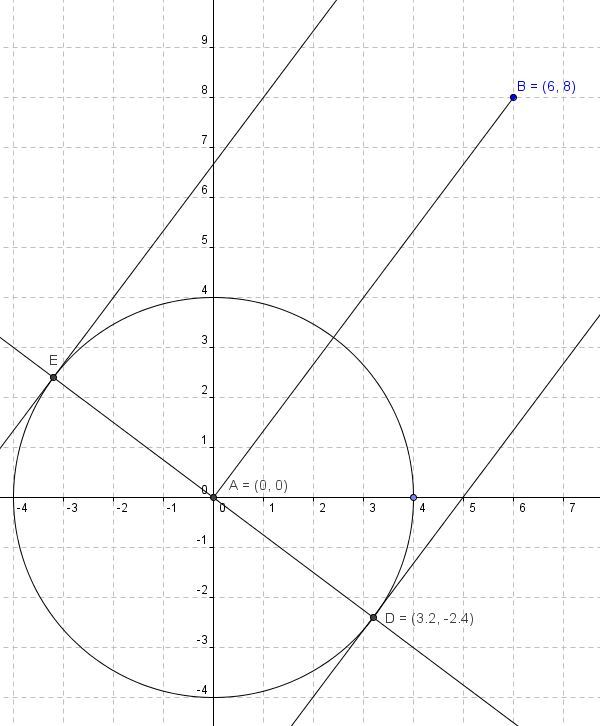# Equal Area Triangles on the Same Base II

Alignments to Content Standards: G-GPE.B.5

1. Given a line segment with end points $A=(0,0)$ and $B=(6,8)$, find all points $C=(x, y)$ such that the triangle with vertices $A$, $B$, $C$ has an area of 20 square units.
2. What mathematical results did you apply to solve this problem?

## IM Commentary

This task has students apply their knowledge of parallel lines to solve a geometric problem on areas of triangles. The task is deliberately open in its directions to students. For example, it is left to students to decide whether to use graph paper, geometry software, or abstract reasoning to complete the task. (Of course, limitations imposed by available resources may be present as well.) Similarly, several different solution techniques present themselves, so teachers should be prepared to provide feedback on a variety of approaches. Between their choice of technique, and the choice of medium for completing the task, the task serves as a good illustration of Standard for Mathematical Practice 5: Use appropriate tool strategically.

Part (b) of the problem has students reflect on both the technique they used, and the key mathematical concepts involved in the problem. There are many ways to extend the problem; e.g. write the equations of the locus solving the problem. It may be interesting to see if and how various student solution strategies would continue to such extensions.

Note that a somewhat more elementary version of this task, possibly useable as a warm-up to the current task, can be found at G-GPE Equal Area Triangles on the Same Base I.

## Solution

1. Since the segment $AB$ has length 10 and the area is 20, we know the height of the triangle must be 4. Here are a number of solutions; the first is geometric, the others more algebraic. Almost all involve the construction of points $D$ and $E$ which are a distance of 4 from $AB$, and then drawing lines through $D$ and $E$ parallel to $AB$.

1. We can construct such points geometrically as follows: Draw a circle with radius 4 at $A$, and erect a perpendicular to $AB$ at $A$. The two intersections of the circle and the perpendicular are our points $D$ and $E$. Note that triangles $\triangle ABD$ and $\triangle ABE$ have area 20, and similarly for any point on the lines through $D$ or $E$ parallel to $AB$. Note that the perpendicular and parallel line can be constructed with computer geometry software, e.g., GeoGebra.

2. We can construct $D$ and $E$ algebraically as follows: The equation of the line through $A$ and $B$ is $y = \frac{4x}{3}$. So the slope of the perpendicular line is $-\frac{3}{4}$, and so the equation of the line though $A$ and $D$ is $y = -\frac{3x}{4}$. Thus each point on the line has the form $(a, -\frac{3a}{4})$. So we know $D$ has coordinates $(a, \frac{-3a}{4})$ and the vertical line through $D$ intersects the $x$-axis at $(a,0)$. But the length of $AD$ is 4. So $a^2 + \frac{(-3a)^2}{4} = 4^2$. That is, $16a^2 +9a^2 = 256$ or $a^2 = \frac{256}{25}$ and $a = \frac{16}{5}$ (since we chose $D$ so that $a$ is positive). And then the $y$-coordinate of $D$ is $-\frac{3}{4} \times \frac{16}{5}$ which is $-\frac{12}{5}$.

3. Here is another way to finish method ii and find the coordinates of $D$. If those coordinates are $(x, y)$ we have two equations:

$$\begin{equation} y = -\frac{3x}{4} \\ x^2 + y^2 = 16 \end{equation}$$

Substitute $-\frac{3x}{4}$ for $y$ in the second equation to get $x^2 + \frac{9}{16}x^2 = 16$. That is, $\frac{25}{16}x^2 = 16$ or $x^2 = \frac{16 \times 16}{25}$ or $x = \pm16$. These are the $x$ coordinates of $E$ and $D$ in the diagram. And the calculation of the $y$-coordinate is as in solution ii.

4. Here is still another way to finish method ii and find the coordinates of $D$. The perpendicular through $(0, 0)$ is $y = -\frac{3x}{4}$ so, any point on that line is of the form $(4a, -3a)$ As the altitude must be 4 to get an area of 20, the distance from $(0, 0)$ to $(4b, -3b)$ is 4. Using Pythagoras, $(4b)^2 + (-3b)^2 = 4^2$ so $b = \frac45$ or $a = -\frac45$. And again the coordinates of $D$ are $(16, -12)$ or $(-16, 12)$. Now we can make parallel lines through both points.5. Here is a quite different approach using a trick often taught in math clubs is the matrix determinant way: The area is plus or minus half the determinant of the 3x3 matrix formed by making the first column the $x$-coordinates, the second column the $y$-coordinates, the third columns all 1s. Evaluating this expression and selecting plus or minus gives the equation of the two different lines of solution.

$$\pm \frac12 \begin{vmatrix} 0 & 0 & 1 \\ 6 & 8 & 1 \\ x & y & 1 \end{vmatrix}$$

So

$$\pm \frac12 (8x - 6y) = 20.$$

Thus $4x - 3y = 20$ and $3y - 4x = 20$ are the equation of the two lines. Any point is either line is a possible choice for $C$.

6. Different solution techniques will require different pieces of content knowledge, but in common to every solution will be the following:
• The formula for the area of a triangle
• The observation that any point on a line parallel to a line through $AB$ is the same distance from that line.
• The Pythagorean theorem to compute the length of the "base" of the triangle.
It is likely that further pieces of information, e.g., that parallel lines have the same slope, slopes of perpendicular lines, geometric constructions of parallel lines, etc., will be used as well.